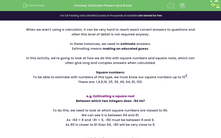# Estimate Powers and Roots

In this worksheet, students will estimate the answers to squared powers and roots so that they are able to work these out without a calculator.Key stage:  KS 4

Year:  GCSE

GCSE Subjects:   Maths

GCSE Boards:   Pearson Edexcel, OCR, Eduqas, AQA,

Curriculum topic:   Number, Indices and Surds

Curriculum subtopic:   Structure and Calculation Powers and Roots

Popular topics:   Estimation worksheets, Square Numbers worksheets

Difficulty level:#### Worksheet Overview

When we aren't using a calculator, it can be very hard to reach exact correct answers to questions and often this level of detail is not required anyway.

In these instances, we need to estimate answers.

Estimating means making an educated guess.

In this activity, we're going to look at how we do this with square numbers and square roots, which can often give long and complex answers when calculated.

Square numbers:

To be able to estimate with numbers of this type, we must know our square numbers up to 102.

These are: 1,4,9,16, 25, 36, 49, 64, 81, 100.

e.g. Estimating a square root

Between which two integers does √80 lie?

To do this, we need to look at which square numbers are closest to 80.

We can see it is between 64 and 81.

As √64 = 8 and √81 = 9, √80 must be between 8 and 9.

As 80 is closer to 81 than 64, √80 will be very close to 9.

e.g. Estimating a square number

Between which two squares will 7.12 lie?

Again, we need to look at our square numbers.

7.1 is between 7 and 8.

72 = 49, 82 = 64

Therefore, 7.12 must be between 49 and 64.

In this activity, we will estimate the answers to squared powers and roots so that we are able to work these out without a calculator.

This will be very useful for your mental maths, speed in solving problems, and checking that your answers are feasible.

### What is EdPlace?

We're your National Curriculum aligned online education content provider helping each child succeed in English, maths and science from year 1 to GCSE. With an EdPlace account you’ll be able to track and measure progress, helping each child achieve their best. We build confidence and attainment by personalising each child’s learning at a level that suits them.

Get started#### Popular Maths topics

••••